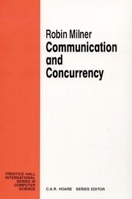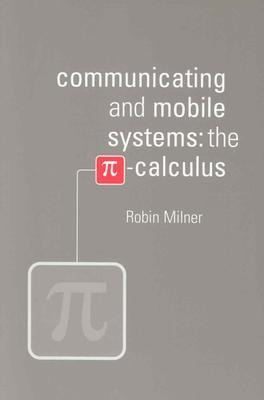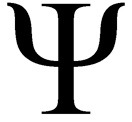## My PhDMy PhD work focussed on formalising the meta-theories of process calculi inside the interactive proof assistant Isabelle. The calculi i formalised were CCS (by Milner), the pi-calculus (by Milner, Parrow, and Walker), and the psi-calculi framework (by myself, Magnusson, Parrow, and Victor).

The advantages of formalising mathematics inside a theorem prover are numerous. Primarily, we know with absolute certainty, at least up to the state of the art of modern theorem provers, that our results are correct. No stone has been left unturned. No obscure corner cases have been forgotten. Another important gain is that once the formalism is in place, it is very easy to make modifications to the theories and immediately see where the proofs break; a paper proof will typically have to be redone from the bottom up and the risk of overlooking some detail during this tedious process is often great.

On this page you can find more detailed information about my formalisation work, as well as links to the development files in the Isabelle Archive of Formal Proofs where they have all been published.

My thesis can be downloaded from here, or from my publication page.

The Calculus of Communicating Systems (CCS)CCS was introduced by Robin Milner around 1980 and was designed to allow modeling of systems composed of components running in parallel and communicating with each other. I formalised the following results from his book Communication and Concurrency.

• strong bisimilarity is a congruence
• strong bisimilarity respects the laws of structural congruence
• weak bisimilarity is preserved by all operators except sum
• weak congruence is a congruence
• all strongly bisimilar agents are also weakly congruent which in turn are weakly bisimilar. As a corollary, weak bisimilarity and weak congruence respect the laws of structural congruence.

The Isabelle sources can be downloaded from the AFP entry CCS in nominal logic.The pi-calculus

The pi-calculus was introduced by Robin Milner, Joachim Parrow, and David Walker in 1992. It extends on CCS by allowing the components of a system to transfer not only data, but also network addresses to itself or to other components. This allows the topology of the network to change while the agents are executing. I formalised the following results, where the notation (e) means that the results cover the early operational semantics and (l) the late one.

• strong bisimilarity is preserved by all operators except the input-prefix (e/l)
• strong equivalence is a congruence (e/l)
• weak bisimilarity is preserved by all operators except the input-prefix and sum (e/l)
• weak congruence is a congruence (e/l)
• strong equivalence respect the laws of structural congruence (l)
• all strongly equivalent agents are also weakly congruent which in turn are weakly bisimilar. Moreover, strongly equivalent agents are also strongly bisimilar (e/l)
• all late equivalences are included in their early counterparts.
• as a corollary of the last three points, all mentioned equivalences respect the laws of structural congruence
• the axiomatisation of the finite fragment of strong late bisimilarity is sound and complete
• the Hennessy lemma

The Isabelle sources can be downloaded from the AFP entry The pi-calculus in nominal logic

Psi-calculiThe Psi-calculi is a parametric framework for process calculi developed in 2009 by myself, Magnus Johansson, Joachim Parrow, and Björn Victor. It is parametric on three components - terms (representing the data that the agents communicate), conditions (representing the conditional tests that can be checked at execution branches), and assertions (representing the knowledge stored in an environment). Intuitively, by properly instantiating these parameters, a user can create an instance of psi-calculi conforming to an already existing process calculus (such as CCS, pi, applied pi, the spi-calculus, etc) or create a completely new calculus. I formalised all of the meta-theory of psi-calculi in Isabelle, and hence the following results will hold for any valid psi-calculus instance. The author of the instance does not have to prove them again. Psi-calculi currently only have early operational semantics. A late semantics may be constructed in the future.

• strong bisimilarity is preserved by all operators except the input-prefix
• strong equivalence is a congruence
• weak bisimilarity is preserved by all operators except case and the input-prefix
• weak congruence is a congruence
• strong equivalence respect the laws of structural congruence
• all strongly equivalent agents are also weakly congruent which in turn are weakly bisimilar. Moreover, strongly equivalent agents are also strongly bisimilar
• as a corollary of the last two points, all mentioned equivalences respect the law of structural congruence
• for instances of psi-calculi where assertion composition satisfies weakening, the definition of weak bisimilarity can be simplified significantly and proven equivalent to the version that applies when weakening does not hold
• for certain versions of psi-calculi, sum can be encoded
• for certain versions of psi-calculi, the tau-prefix can be encoded and when weakening is satisfied, all of the tau-laws hold.

The Isabelle sources can be downloaded from the AFP entry Psi-calculi in Isabelle.